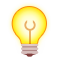## 杭电多校第四场

August 2, 2020 · 算法 · 719次阅读

# 1004 Deliver the Cake

1
3 3 1 3 100
LRM
1 2 10
2 3 10
1 3 100

100

## 代码（还是有点问题的代码）

``````#include <iostream>
#include <cstring>
#include <algorithm>
#include <queue>
#include <vector>

using namespace std;
typedef long long ll;

typedef pair<int, int> pi;
const int maxn = 200000 + 10;
const ll inf = 1e18;
int T;
int n, m, s, t, x;
ll dis[maxn];
char type[maxn];
struct Edge { int v, w; };
vector<Edge> vc[maxn];
struct Node {
ll d;//该节点的代价
int u, t;//u是节点编号 t是节点类型（L/R）

bool operator<(const Node &b) const { //重载运算符用于对pair排序，优先队列从小到大
return d > b.d; //这里的符号和优先队列最终排序的顺序是相反的
}
};

priority_queue<Node> pq;

void solve() {
cin >> n >> m >> s >> t >> x;
cin >> (type+1);
for (int i = 1; i <= m; i++) {
int u, v, d;
cin >> u >> v >> d;
vc[u].push_back(Edge{v, d});
vc[v].push_back(Edge{u, d});
}
for (int i = 1; i <= n; i++) dis[i] = dis[i] = inf;
if (type[s] != 'R') pq.push(Node{dis[s] = 0, s, 0});//将出发节点加入队列 同时判断出发节点左右手
if (type[s] != 'L') pq.push(Node{dis[s] = 0, s, 1});//这里用!=是因为要把M这个类型搞进去
while (!pq.empty()) {
Node now = pq.top();
pq.pop();
if (dis[now.u][now.t] < now.d) continue;//如果这个点已经被更新过了就不用管了
if (now.u == t) break;//到达终点
for (const Edge &e:vc[now.u]) {
ll w = e.w + now.d;//总代价等于现在选的路的代价+目前为止的总代价
int tt = type[e.v] == 'R' ? 1 : (type[e.v] == 'L' ? 0 : now.t);//如果不是左边也不是右边。就和上一个点保持一致
if (tt == now.t && w < dis[e.v][tt]) {//若走这条路不需要换手
pq.push(Node{dis[e.v][tt] = w, e.v, tt});
}
w += x;
if (type[e.v] == 'M') t = !t;//M这个可以换手也可以不换，不换的上一个if考虑到了，现在考虑换手
if (tt != now.t && w < dis[e.v][tt]) {
pq.push(Node{dis[e.v][tt] = w, e.v, tt});
}
}
}
cout << min(dis[t], dis[t]) << endl;
while (!pq.empty()) pq.pop();
for (int i = 1; i <= n; i++) vc[i].clear();//init
}

int main() {
for (cin >> T; T--;) solve();
return 0;
}``````

# 1005 Equal Sentences

## 样例输入

2
6
he he zhou is watching you
13
yi yi si wu yi si yi jiu yi jiu ba yao ling

8
233

## AC代码

``````#include <iostream>
#include <cstring>
#include <algorithm>

using namespace std;
const int maxn = 100000 + 10;
const int mod = 1e9 + 7;
int t;
int f[maxn];
string s[maxn];

int solve() {
int n;
memset(f, 0, sizeof(f));
f = 1;//加上其本身
cin >> n;
for (int i = 1; i <= n; i++) cin >> s[i];
for (int i = 1; i <= n; i++) {
f[i] = f[i - 1];//继承上一单词为止的相似总数
if (i >= 2 && s[i] != s[i - 1]) {
f[i] += f[i - 2];//i-2是因为要加上上一阶段（上一次交换）前的字符串结果
f[i] %= mod;
}
}
return f[n];
}

int main() {
for (cin >> t; t--;) cout << solve() << endl;
return 0;
}``````

# 1011 Kindergarten Physics

3
1 2 3 4
7 73 7 68
100 100 1 100

## 样例输出

2.99999999999999999982
6.99999999999999974807
0.99999999999993325700

## AC代码（STD）

``````#include <cstdio>
#include <cstring>
#include <algorithm>
using namespace std;

int main(void) {
int T;
scanf("%d", &T);
while (T--) {
int d;
scanf("%*d%*d%d%*d", &d);
printf("%d\n", d);
}
return 0;
}``````## 添加新评论

•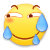•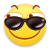•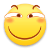•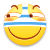•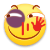•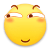•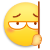•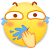•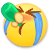•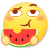•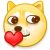•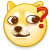•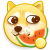••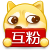•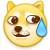•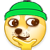•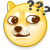•••••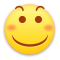•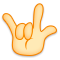•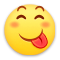•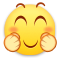•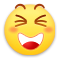•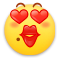•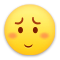•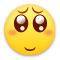•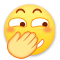•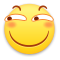•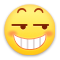••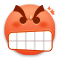•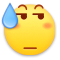•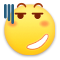•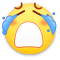•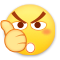•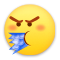••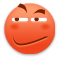•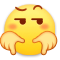•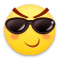•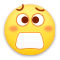•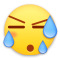•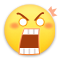•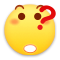•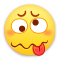•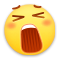•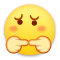•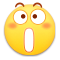•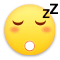•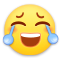•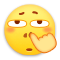•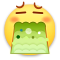•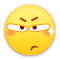•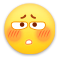•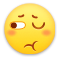•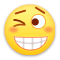•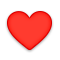•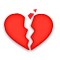•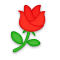•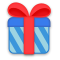•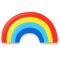•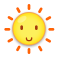•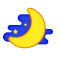•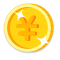•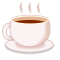•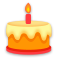•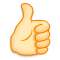•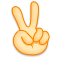••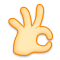•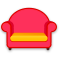•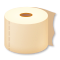•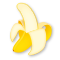•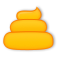•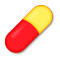•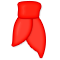•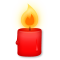•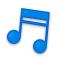•